# MA2051 - Ordinary Differential EquationsReview For Exam II - Solutions

REVIEW QUESTIONS

1. This problem analyzes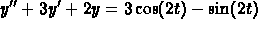.
(a)
The characteristic equation isand so the roots are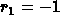and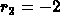. This gives two solutionsand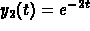. To check independence, compute the Wronskian and show that it is never zero.

(b)
To find a particular solution, use the method of undetermined coefficients and look for a solution of the form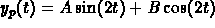. Plug this into the original equation and solve for A and B to obtain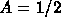and B=0. Hence, a particular solution is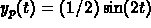.

(c)
The general solution is. The initial data give you equations for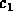and. Solving these equations give the solution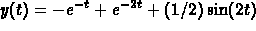.

(d)
The transient component is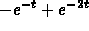(because this is the part that converges to zero as t goes to infinity). This is less thanfor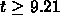. (Just set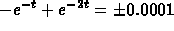and solve for t; it is a quadratic equation for.)

(e)
The limiting periodic solution (obtained in part (c)) is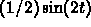. The period is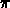and the amplitude is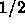.

(f)
Go back to the general solution in part (c) and choose the initial data so that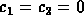. The right choice is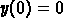and.

2. The characteristic equation has roots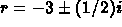, so that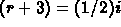. Square both sides (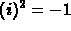) and simplify to obtain the characteristic equation. It follows that the original differential equation was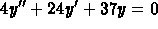.

For part (b),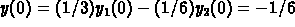. Similarly, with a little differentiation,.

3. This problem analyzes a spring-mass system.
(a)
The system is undamped and there is no forcing term, so the mathematical model that you want is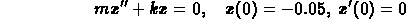where m = 6 and k = 98.

(b)
The general solution is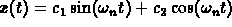where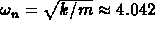is the natural frequency and the period isseconds.

To find the amplitude of the motion, use the initial data to solve forand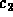to obtain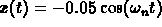. The amplitude ismeters.

(c)
The period is. If you increase the mass by a factor of 4, you will double the period.

The amplitude will not change with a change in the mass. (It depends only on the initial position in this problem.)

(d)
The new model is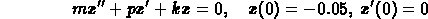and the system will exhibit oscillations as long as the characteristic equation has complex roots. This occurs for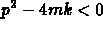. Plug in the numbers to find that you have oscillations for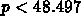.

4. Solve a system of first-order equations.
(a)
The characteristic equation isThe roots are(where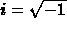). The corresponding solution pairs are(You obtainfrom, for example, by substituting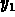back into the first differential equation in the system.) To verify independence, compute the Wronskian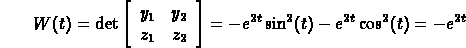The Wronskian is never zero, so the solutions are linearly independent. The general solution pair is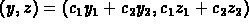whereandare arbitrary constants.

(b)
The initial data give you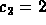and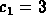in the formula for the general solution.

(c)
Plot a few points to obtain a spiral heading away from the origin.

(d)
Differentiate the quantity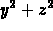to obtainThis last quantity is always positive and so, as soon as the solution curve is away from the stationary point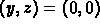, its distance from the origin is strictly increasing.

5. The first step is to write the second-order equation as a first-order system:Euler's method starts withand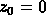(the initial data). Because you want the solution at t=1 and you are using two steps,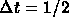. The next two steps are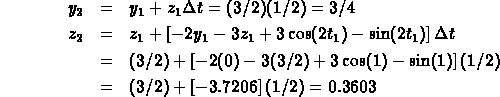Your approximation for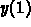is. From Problem #1, the exact value is. The absolute error is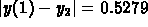. The relative error is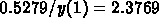, or about 237%. (Bigerror.)

```© 1996 by Will Brother.
All rights Reserved. File last modified on April 25, 1996.

```